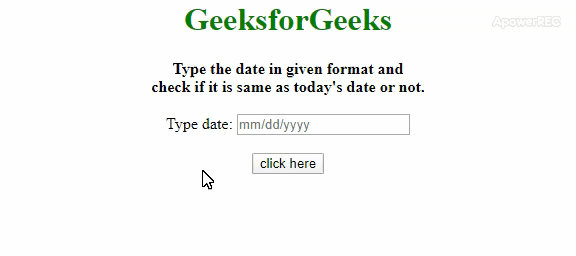# How to check the input date is equal to today’s date or not using JavaScript ?

Given a date object and the task is to determine whether the given date is the same as today’s date or not with the help of JavaScript. There are two well-famous approaches are discussed here.

Approach 1: Get the input date from user (var inpDate) and the today’s date by new Date(). Now, use .setHours() method on both dates by passing parameters of all zeroes. All zeroes are passed to make all hour, min, sec and millisec to 0. Now compare today’s date with given date and display the result.

• Example: This example implements the above approach.

 `  ` `<``html``>  ` ` `  `<``head``>  ` `    ``<``title``>  ` `        ``How to Check Input Date is Equal ` `        ``to Today’s Date or not using ` `        ``JavaScript? ` `    ``  ` `     `  `    ``<``style``> ` `        ``body { ` `            ``text-align: center; ` `        ``} ` `        ``h1{ ` `            ``color: green; ` `        ``} ` `        ``#geeks { ` `            ``color: green;  ` `            ``font-size: 29px;  ` `            ``font-weight: bold; ` `        ``} ` `    `` ` `  ` ` `  `<``body` `>  ` `    ``<``h1``>GeeksforGeeks ` `     `  `    ``<``b``> ` `        ``Type the date in given format ` `        ``and <``br``>check if it is same as ` `        ``today's date or not. ` `    `` ` `     `  `    ``<``br``><``br``> ` `     `  `    ``Type date: <``input` `id` `= ``"date"` `            ``placeholder``= ``"mm/dd/yyyy"``/> ` `    ``<``br``><``br``> ` `     `  `    ``<``button` `onclick` `= ``"gfg();"``> ` `        ``click here ` `    `` ` `     `  `    ``<``p` `id` `= ``"geeks"` `> ` `     `  `    ``<``script``> ` `        ``var down = document.getElementById('geeks'); ` `         `  `        ``function gfg() { ` `            ``var date =  ` `                ``document.getElementById('date').value; ` `             `  `            ``var inpDate = new Date(date); ` `            ``var currDate = new Date(); ` `             `  `            ``if(inpDate.setHours(0, 0, 0, 0) ==  ` `                    ``currDate.setHours(0, 0, 0, 0)) ` `            ``{ ` `                ``down.innerHTML =  ` `                    ``"The input date is today's date"; ` `            ``}  ` `            ``else { ` `                ``down.innerHTML = "The input date is" ` `                    ``+ " different from today's date"; ` `            ``}          ` `        ``} ` `    ``  ` `  ` ` `  `          `

• Output:Approach 2: Similarly get the input date from user (var inpDate) and the today’s date by using new Date(). Now, we will use .toDateString() method on both dates to convert them to readable strings. Now compare today’s date with given date and display the result.

• Example: This example implements the above approach.

 `  ` `<``html``>  ` ` `  `<``head``>  ` `    ``<``title``>  ` `        ``How to Check Input Date is Equal ` `        ``to Today’s Date or not using ` `        ``JavaScript? ` `    ``  ` `     `  `    ``<``style``> ` `        ``body { ` `            ``text-align: center; ` `        ``} ` `        ``h1 { ` `            ``color: green; ` `        ``} ` `        ``#geeks { ` `            ``color: green;  ` `            ``font-size: 29px;  ` `            ``font-weight: bold; ` `        ``} ` `    `` ` `  ` ` `  `<``body` `>  ` `    ``<``h1``>GeeksforGeeks ` `     `  `    ``<``b``> ` `        ``Type the date in given format ` `        ``and <``br``>check if it is same as ` `        ``today's date or not. ` `    `` ` `     `  `    ``<``br``><``br``> ` `     `  `    ``Type date: <``input` `id` `= ``"date"` `            ``placeholder``= ``"mm/dd/yyyy"``/> ` `    ``<``br``><``br``> ` `     `  `    ``<``button` `onclick` `= ``"gfg();"``> ` `        ``click here ` `    `` ` `     `  `    ``<``p` `id` `= ``"geeks"``> ` `     `  `    ``<``script``> ` `        ``var down = document.getElementById('geeks'); ` `         `  `        ``function gfg() { ` `            ``var date =  ` `                ``document.getElementById('date').value; ` `             `  `            ``var inpDate = new Date(date); ` `            ``var currDate = new Date(); ` `             `  `            ``if(currDate.toDateString() ==  ` `                        ``inpDate.toDateString())  ` `            ``{ ` `                ``down.innerHTML =  ` `                    ``"The input date is today's date"; ` `            ``} ` `            ``else { ` `                ``down.innerHTML = "The input date is" ` `                        ``+ " different from today's date"; ` `            ``} ` `        ``} ` `    ``  ` `  ` ` `  ` `

• Output:My Personal Notes arrow_drop_upCheck out this Author's contributed articles.

If you like GeeksforGeeks and would like to contribute, you can also write an article using contribute.geeksforgeeks.org or mail your article to contribute@geeksforgeeks.org. See your article appearing on the GeeksforGeeks main page and help other Geeks.

Please Improve this article if you find anything incorrect by clicking on the "Improve Article" button below.